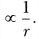# AB is a current-carrying conductor in the plane of the paper as shown in figure. What are the directions of magnetic fields produced by it at points P and Q? Given r1 > r2, where will the strength of the magnetic field be larger?

AB is a current-carrying conductor in the plane of the paper as shown in figure. What are the directions of magnetic fields produced by it at points P and Q? Given r1 > r2, where will the strength of the magnetic field be larger?Magnetic field at P is into the plane of paper and at Q it is out of the plane of paper. The strength of the magnetic field at Q will be larger as strength of the field